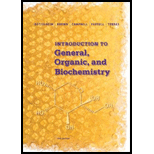# 6-15 Answer true or false. (a) A solute is the substance dissolved in a solvent to form a solution. (b) A solvent is the medium in which a solute is dissolved to form a solution. (c) Some solutions can be separated into their components by filtration. (d) Acid rain is a solution.BuyFind

### Introduction to General, Organic a...

11th Edition
Frederick A. Bettelheim + 4 others
Publisher: Cengage Learning
ISBN: 9781285869759BuyFind

### Introduction to General, Organic a...

11th Edition
Frederick A. Bettelheim + 4 others
Publisher: Cengage Learning
ISBN: 9781285869759

#### Solutions

Chapter 6, Problem 6.15P
Textbook Problem

## 6-15 Answer true or false. (a) A solute is the substance dissolved in a solvent to form a solution. (b) A solvent is the medium in which a solute is dissolved to form a solution. (c) Some solutions can be separated into their components by filtration. (d) Acid rain is a solution.

Expert Solution
Interpretation Introduction

Interpretation: Whether the given statements are true or false should be determined.

Concept introduction:

• Solute: The component which is present in less amount in solution.
• Solvent: The component which is present in greater amount in solution.
• Solution: Solution is the homogeneous mixture of solute and solvent.
• Filtration: It is the process through which two mixtures having small particles which can be seen through naked eyes can be separated.

## Answer to Problem 6.15P

All statements are true.

### Explanation of Solution

Reason for true statements:

1. The given statement is “solute is a substance that dissolve in solvent to form a solution”. According to the definition of solution, it is the homogeneous mixture of solute and solvent. So, solute can be mixed with solvent to form a solution.
2. The given statement is “a solvent is the medium in which a solute is dissolved to form a solution”. According to the definition of solution, it is the homogeneous mixture of solute and solvent. So, solvent is the medium in which a solute is dissolved to form a solution.
3. The given statement is “some solutions can be separated into their components by filtration”. According to the definition of filtration, the solution having particles which can be seen through naked eyes can be separated like chalk powder solution.
4. The given statement is “acid rain is a solution”. According to the definition of solution, it is the homogeneous mixture of solute and solvent. Acid rain is the mixture of different solute and solvent in the form of acid and water.
Conclusion

Therefore, all the statements are true.

### Want to see more full solutions like this?

Subscribe now to access step-by-step solutions to millions of textbook problems written by subject matter experts!

### Want to see more full solutions like this?

Subscribe now to access step-by-step solutions to millions of textbook problems written by subject matter experts!© 2021 bartleby. All Rights Reserved.